# QUADRATIC EQUATION - INTRODUCTION , SOLUTION OF QUADRATIC EQUATION & RELATION BETWEEN ROOTS & CO-EFFICIENTS

INTRODUCTION

The algebraic expression of the form ax2 + bx + c, a  0 is called a quadratic expression, because the highest order term

in it is of second degree. Quadratic equation means, ax2 + bx + c = 0. In general whenever one says zeroes of the

expression ax2+bx + c, it implies roots of the equation ax2 + bx + c = 0, unless specified otherwise. A quadratic

equation has exactly two roots which may be real (equal or unequal) or imaginary.

SOLUTION OF QUADRATIC EQUATION & RELATION BETWEEN ROOTS & CO-EFFICIENTS

a.

The general form of quadratic equation is ax2 + bx + c = 0, a != 0.

The roots can be found in following manner :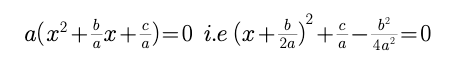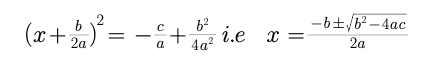This expression can be directly used to find the two roots of a quadratic equation

b.

The expression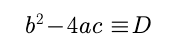is called the discriminant of the quadratic equation.

c.

if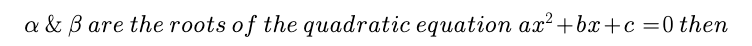1.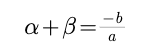2.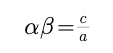3.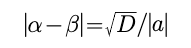d.A quadratic equation whose roots are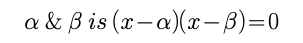Example :

if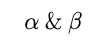are  the roots of a quadratic equation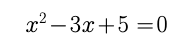, then the equation whose roots are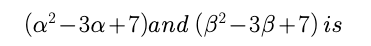Solution :

sinceare  the roots of a quadratic equationso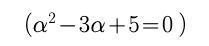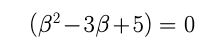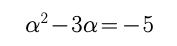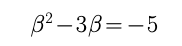Putting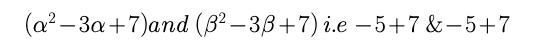2 and 2 are the roots.

The required equation is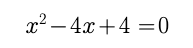Post By : Vivek Tiwari 02 Apr, 2020 1955 views Maths